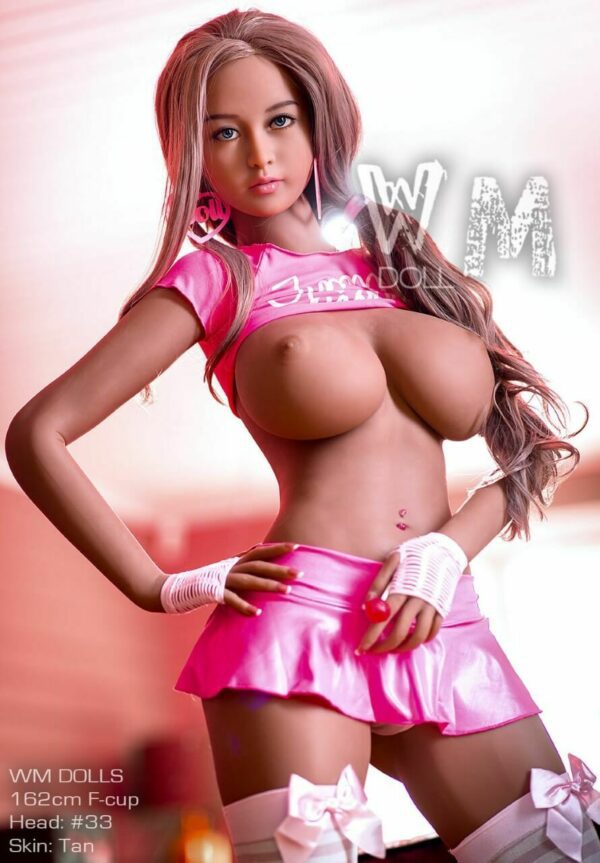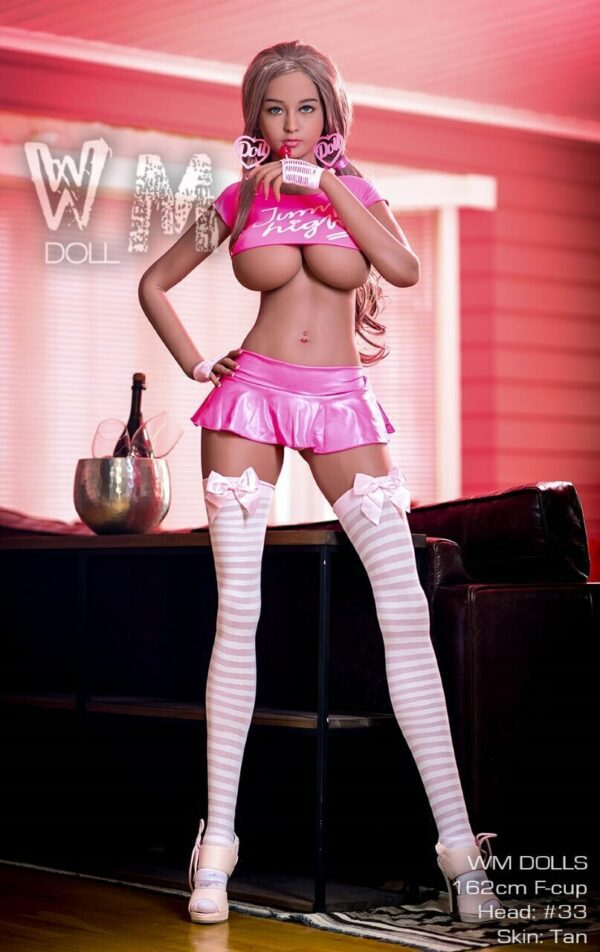# Galenka – 5’4″ | 162cm Teen Sex Doll

WM Doll
\$1,700

Galenka – 5’4″ | 162cm Teen Sex Doll made with high quality and safety standards by WM Doll, this genuine silicone and TPE sexy real sex doll is CE certified. With various features complementing each other, this realistic sex doll is sure to please the owner.

## Description

Height > 5ft 3.5 in – 5ft 5in (161cm – 165cm), Ethnicity > American, Best Sellers, Body Style > Curvy, Age > Eighteen & Nineteen, Ethnicity > European, Material > Fully TPE, Ethnicity > Interracial, Breast Size > Large, Hair Color > Redhead, See All Sex Dolls, Body Style > Slender, BRANDS > WM Doll, Age > Young Adult

• \$
• \$
• \$
• \$
• \$
• \$
• \$
• \$
• \$
• \$
• \$
• \$
• \$
• \$
• \$
• \$
• \$
• \$
• \$
• \$
• \$
• \$
• \$
• \$
• \$
• \$
• \$
• \$
• \$
• \$
• \$
• \$
• \$
• \$
• \$
• \$
• \$
• \$
• \$
• \$
• \$
• \$
• \$
• \$
• \$
• \$
• \$
• \$
• \$
• \$
• \$
• \$
• \$
• \$
• \$
• \$
• \$
• \$
• \$
• \$
• \$
• \$
• \$
• \$
• \$
• \$
• \$
• \$
• \$
• \$
• \$
• \$
• \$
• \$
• \$
• \$
• \$
• \$
• \$
• \$
• \$
• \$
• \$
• \$
• \$
• \$
• \$
• \$
• \$
• \$
• \$
• \$
• \$
• \$
• \$
• \$
• \$
• \$
• \$
• \$
• \$
• \$
• \$
• \$
• \$
• \$
• \$
• \$
• \$
• \$
• \$
• \$
• \$
• \$
• \$
• \$
• \$
• \$
• \$
• \$
• \$
• \$
• \$
• \$
• \$
• \$
• \$
• \$
• \$
• \$
• \$
• \$
• \$
• \$
• \$
• \$
• \$

• \$
• \$
• \$
• \$
• \$
• \$
• \$

• \$

• \$
• \$
• \$
• \$
• \$
• \$
• \$
• \$
• \$
• \$
• \$
• \$
• \$
• \$
• \$
• \$
• \$
• \$
• \$
• \$
• \$

• \$
• \$
• \$
• \$
• \$
• \$
• \$
• \$
• \$
• \$
• \$
• \$
• \$
• \$
• \$
• \$
• \$
• \$
• \$
• \$

• \$
• \$
• \$
• \$
• \$
• \$
• \$

• \$
• 40 \$

• \$
• 100 \$

• \$
• \$
• \$
• \$
• \$

• \$
• \$
• \$
• \$
• \$

• \$
• \$
• \$

• \$
• 130 \$

• \$
• \$
• \$

• \$
• 40 \$

• \$
• \$
• \$
• \$

• \$
• \$
• \$
• \$
• \$

• \$
• \$

• \$
• \$
• \$
• \$
• \$
• \$
• \$
• \$
• \$
• \$

• \$
• \$
• \$
• \$
• \$
• \$

• \$
• 200 \$

• \$
• 100 \$

• \$
• 675 \$

• \$
• 390 \$

## Doll Measurements

Height: 162 cm | 5 ft 3.7 in
Weight: 33.3kg | 73.4 lbs.
Shoulder: 35 cm | 13.8 in
Full Bust: 83 cm | 32.6 in
Under Bust: 56 cm | 22 in
Cup Size: F
Waist: 54 cm | 21.2 in
Hips: 87 cm | 34.2 in
Foot Length: 22 cm | 8.2 in
Vaginal Depth: 18cm | 7 in
Anal Depth: 15 cm | 5.9 in
Oral Depth: 12 cm | 4.7 cm